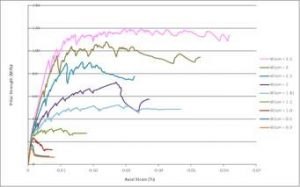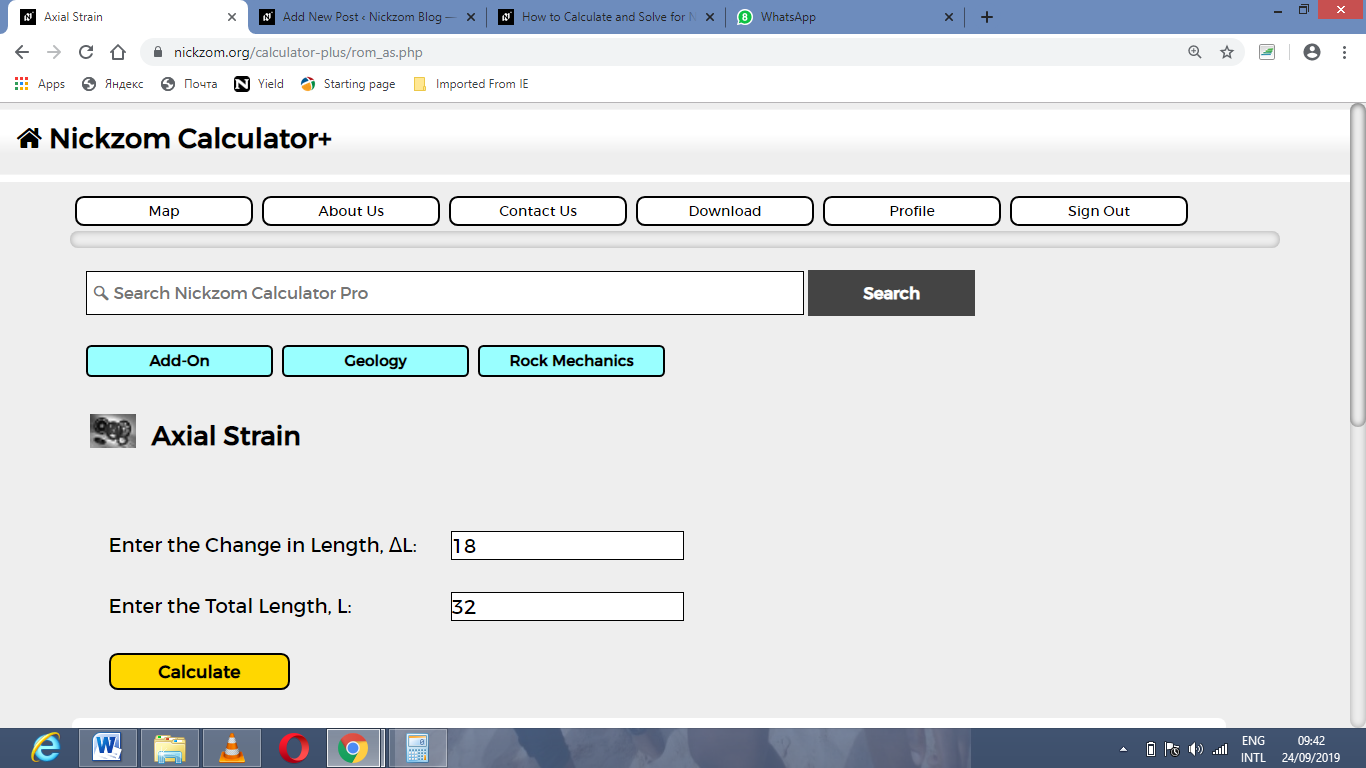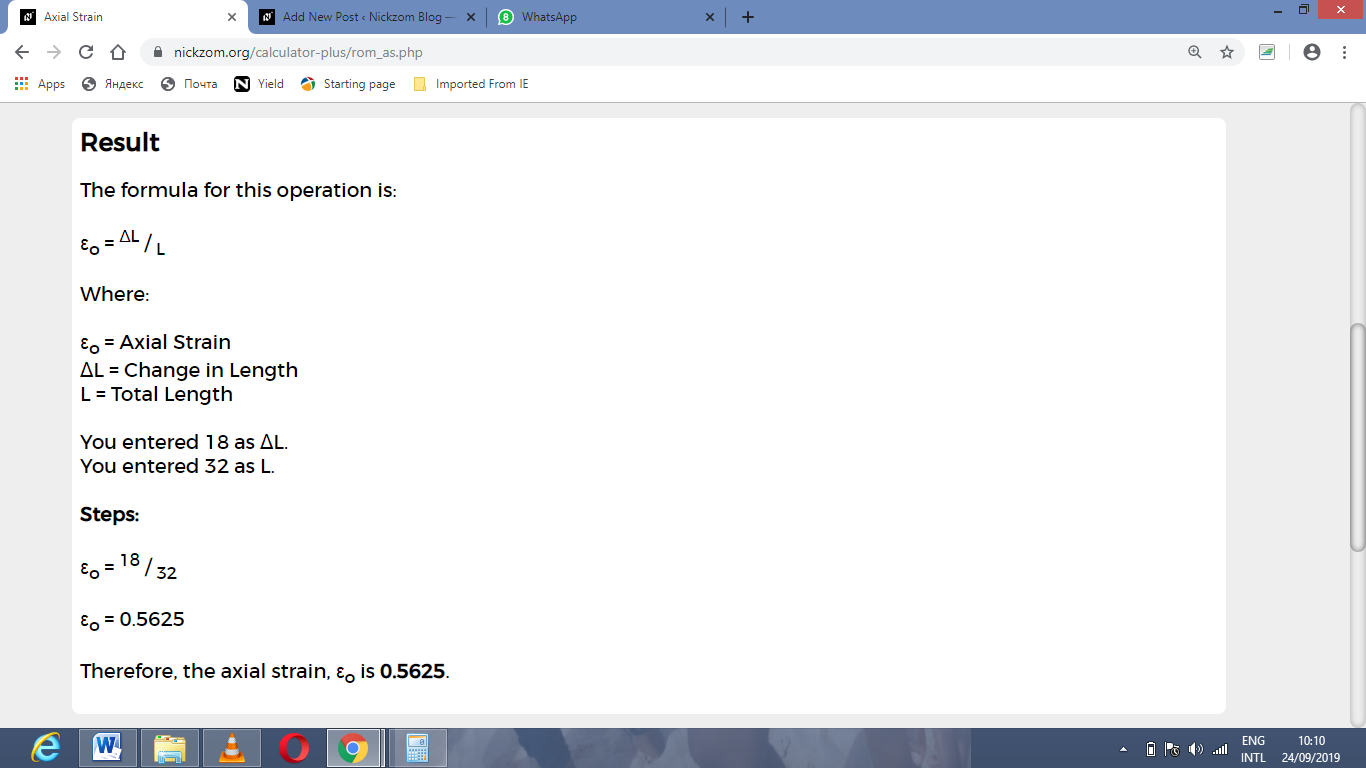# How to Calculate and Solve for Axial Strain | Rock MechanicsThe image above represents axial strain.

To compute for axial strain, two essential parameters are needed and these parameters are change in length (ΔL) and length (L).

The formula for calculating the axial strain:

εo = ΔL / L

Where;

εo = Axial Strain
ΔL = Change in Length
L = Total Length

Let’s solve an example;
Calculate the axial strain when the change in length is 18 and total length is 32.

This implies that;

ΔL = Change in Length = 18
L = Total Length = 32

εo = ΔL / L
εo = 18 / 32
εo = 0.5625

Therefore, the axial strain is 0.5625.

Calculating the Change in Length when the Axial Strain and Total Length is Given.

ΔL = εo x L

Where;

ΔL = Change in Length
εo = Axial Strain
L = Total Length

Let’s solve an example;
Calculate the change in length when the axial strain is 21 and total length is 9

This implies that;

εo = Axial Strain = 21
L = Total Length = 9

ΔL = εo x L
ΔL = 21 x 9
ΔL = 189

Therefore, the change in length is 189.

Calculating the Total Length when the Axial Strain and Change in Length is Given.

L = ΔL / εo

Where;

L = Total Length
εo = Axial Strain
ΔL = Change in Length

Let’s solve an example;
Calculate the total length when the axial strain is 32 and change in length is 60.

This implies that;

εo = Axial Strain = 32
ΔL = Change in Length = 60

L = ΔL / εo
L = 60 / 32
L = 1.875

Therefore, the total length is 1.875.

Nickzom Calculator – The Calculator Encyclopedia is capable of calculating the axial strain.

To get the answer and workings of the axial strain using the Nickzom Calculator – The Calculator Encyclopedia. First, you need to obtain the app.

You can get this app via any of these means:

To get access to the professional version via web, you need to register and subscribe for NGN 1,500 per annum to have utter access to all functionalities.
You can also try the demo version via https://www.nickzom.org/calculator

Apple (Paid) – https://itunes.apple.com/us/app/nickzom-calculator/id1331162702?mt=8
Once, you have obtained the calculator encyclopedia app, proceed to the Calculator Map, then click on Geology under Add-on.Now, Click on Rock Mechanics under GeologyNow, Click on Axial Strain under Rock MechanicsThe screenshot below displays the page or activity to enter your values, to get the answer for the axial strain according to the respective parameters which are the change in length (ΔL) and length (L).Now, enter the values appropriately and accordingly for the parameters as required by the change in length (ΔL) is 18 and length (L) is 32.Finally, Click on CalculateAs you can see from the screenshot above, Nickzom Calculator– The Calculator Encyclopedia solves for the axial strain and presents the formula, workings and steps too.